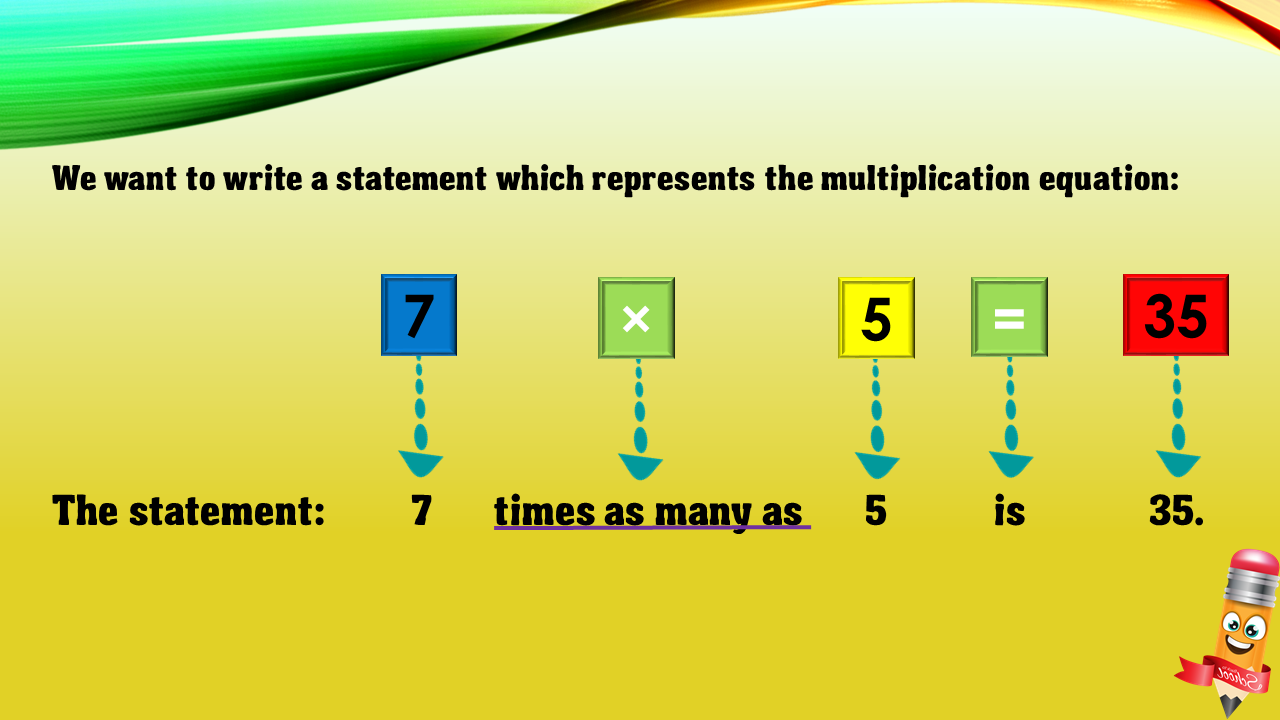1
visibility

Choose one statement which represents the equation:
7 × 5 = 35 ?

• A

7 more than 5 is 35.

• B

7 times as many as 5 is 35.

• C

5 less than 35 is 7.

• D

5 times as many as 35 is 7.